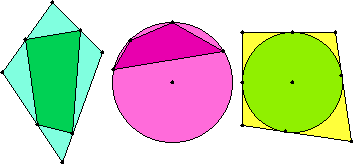# Definition 1

A rectilinear figure is said to be inscribed in a rectilinear figure when the respective angles of the inscribed figure lie on the respective sides of that in which it is inscribed.

# Definition 2

Similarly a figure is said to be circumscribed about a figure when the respective sides of the circumscribed figure pass through the respective angles of that about which it is circumscribed.

# Definition 3

A rectilinear figure is said to be inscribed in a circle when each angle of the inscribed figure lies on the circumference of the circle.

# Definition 4

A rectilinear figure is said to be circumscribed about a circle when each side of the circumscribed figure touches the circumference of the circle.

# Definition 5

Similarly a circle is said to be inscribed in a figure when the circumference of the circle touches each side of the figure in which it is inscribed.

# Definition 6

A circle is said to be circumscribed about a figure when the circumference of the circle passes through each angle of the figure about which it is circumscribed.

# Definition 7

A straight line is said to be fitted into a circle when its ends are on the circumference of the circle.

## Guide

These definitions are used throughout Book IV.Definitions 1 and 2: The first figure shows a smaller quadrilateral inscribed in a larger quadrilateral, and the larger one is circumscribed about the smaller one.

Definitions 3 and 6: The second figure shows a quadrilateral inscribed in a circle, and the circle is circumscribed about the quadrilateral.

Definitions 4 and 5:The third figure shows a circle inscribed in a quadrilateral, and the quadrilateral is circumscribed about the circle.

Definition 7: In the second figure, each side of the quadrilateral is fitted into the circle.